## Capillary action between two glass plates

### Experiment number : 2080

• #### Goal of experiment

We show with this experiment what shape takes the surface of water in a wedgy hollow between two glass plates. In this way we demostrate the dependence of the height water column risen up by capillary action in a capillary tube on the diameter of the tube.

• #### Theory

Imagine that the wedgy space between the glass plates that form a small angle is filled with a series of vertical capillary tubes whose radius r increases with distance from the edge where the glasses touch.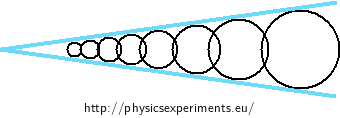The height h of water surface in a capillary tube is given as

$h = \frac{2σ}{rρg},\tag{1}$

where σ is the surface tension of water, r is the inner radius of the tube and ρ os the density of water. It follows from (1) that the height of water in a capillary tube is inversely proportional to the radius of the tube. The radius of the imaginary capillary tubes in the wedge-shaped space between the glass sheets increases with distance x from the edge where the glass plates touch. The height of water in the slit thus decreases with increasing distance from the touch-edge.

The height of water between the glasses can be described with the formula:

$h = \frac{1}{x}·\frac{2σ}{ρgsin\frac{ε}{2}}.\tag{2}$

The following plot shows the theoretical shape of water surface between the glass sheets (2) as a function of distance from the edge where the glass touch.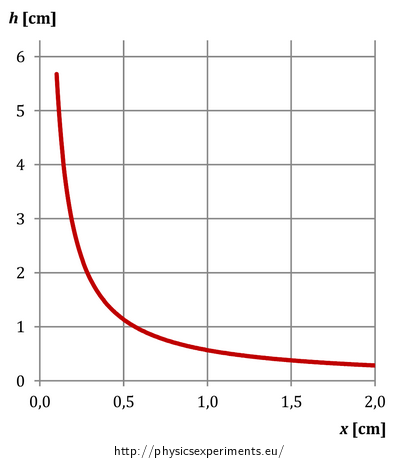Derivation of the dependence of the diameter of the imaginary tubes on the distance from the touching edge and of the height of water on the radius of the tube can be found in the task Capillary action between glass plates.

• #### Equipment

• two glass plates

• open water container (glass with a flat bottom at best)

• water

• food colouring or other water colour

• toothpick or another thin spacer

• rubber band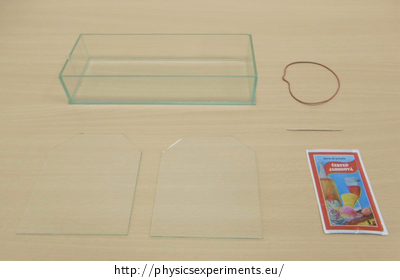• #### Procedure

1. Pour some water in the container (to a height of about 1 cm) and colour it with the colouring.

2. Degrase the glass plates. Place the spacer (toothpick) between the sheets of glass at one edge and clamp the sheets tightly together at the opposite edge. Fix this glass wedge together with the rubber band.

3. Put the side of the wedge in water and observe how high the water rises in the slit as a function of distance from the edge where the glass plates touching each other.

• #### Example of results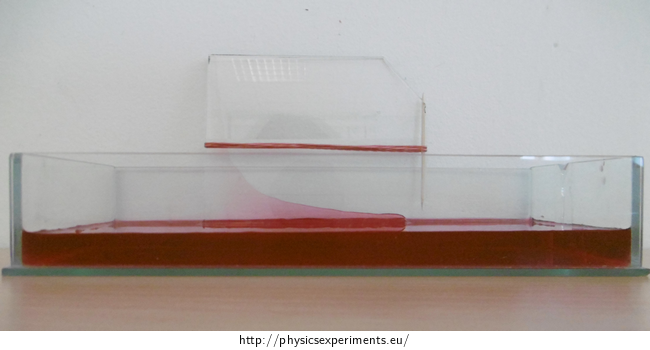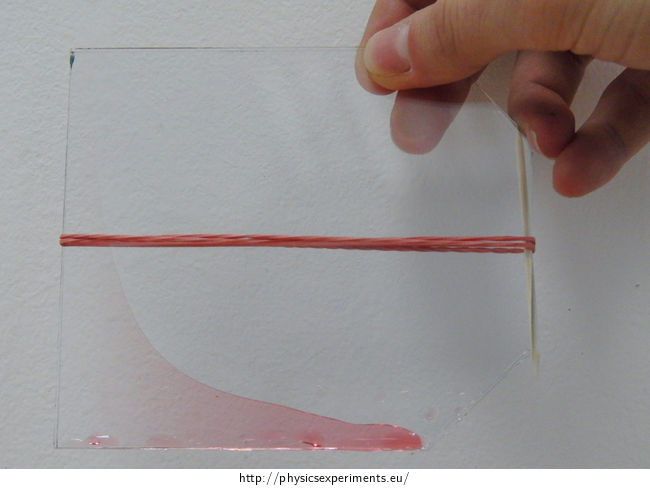• #### Technical notes

We can put the glass wedge out of water after a while and the coloured water will remain in the slit thanks to its adhesion to glass.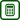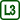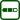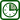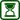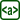×Original source: Svoboda, E. a kol.: Pokusy z fyziky na střední škole 2. Prometheus, Praha 1997